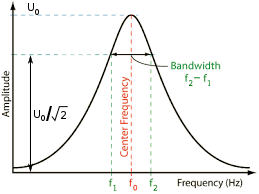# Bandwidth

For a fixed forcing function of magnitude $$F$$, a relative maximum amplitude $$U_0$$ of the system occurs at the resonant frequency $$f_0$$. The amplitude is lower on either side of the resonant frequency. On the low side of the resonant frequency $$f_0$$ there is a frequency $$f_1$$ where the amplitude is only $$U_0 / \sqrt{2}$$. Similarly, on the high side of the resonant frequency $$f_0$$ there is a frequency $$f_2$$ where the amplitude is only $$U_0 / \sqrt{2}$$. Frequencies $$f_1$$ and $$f_2$$ are called the half-power frequencies (i.e., the frequencies where the power will have dropped by half compared to the power at $$f_0$$ at maximum amplitude $$U_0$$).

The bandwidth Bw (in Hz) is defined as:

\begin{align} \label{eq:11801a} Bw = f_2 - f_1 \end{align}Figure 1. Bandwidth

The bandwidth is related to the Q (quality factor) by —

\begin{align} \label{eq:11802a} Q = \frac{f_0}{Bw} \end{align}

Hence, a narrow bandwidth indicates a high Q.

Notes:

1. A complex system (such as an ultrasonic stack) may have many resonances. A separate bandwidth (and associated Q) can be determined for each resonance.
2. For a system that includes piezoelectric ceramics, the amplitude curve of figure 1 becomes more complicated because of the electro-mechanical effect of the ceramics.